# Texas Go Math Grade 2 Lesson 6.2 Answer Key Use Compensation

Refer to our Texas Go Math Grade 2 Answer Key Pdf to score good marks in the exams. Test yourself by practicing the problems from Texas Go Math Grade 2 Lesson 6.2 Answer Key Use Compensation.

## Texas Go Math Grade 2 Lesson 6.2 Answer Key Use Compensation

Explore

Draw quick pictures to show the problems.

FOR THE TEACHER • Have children draw quick pictures to solve this problem. Kara has 47 stickers. She buys 20 more stickers, How many stickers does she have now? Repeat for this problem. 1vrone has 30 stickers and buys 52 more stickers. How many stickers does he have now?
47 + 20 = 67
30 + 52 = 82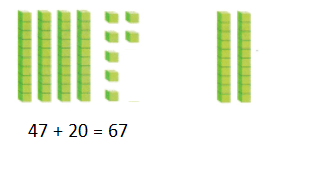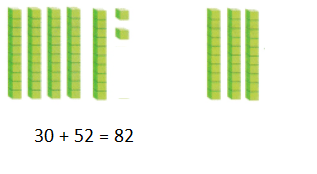Explanation:
The tens and ones are added separately
40 + 7  + 20 =
60 + 7 = 67
Kara has 67
30 + 50 + 2 =
80 + 2 = 82
Tyrone  has 82

Math Talk
Mathematical Processes

Describe how you found how many stickers Tyrone has.
Tens and ones are added separately
30 +52 =
30 +50 + 2 =
80 + 2 = 82

Model and Draw

Take ones from an addend to make the other addend the next tens number.
Adding can be easier when one of the addends is a tens number.
25 + 48 = ?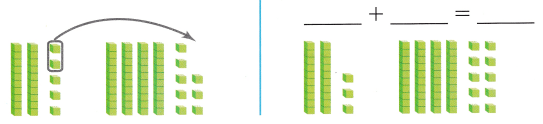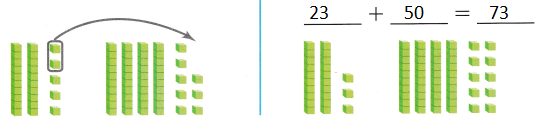Explanation:
25 + 48 = 73
23 + 2 = 25
25 is written as 23 + 2

Share and Show

Show how to make one addend the next tens number. Complete the new addition sentence.

Question 1.
37 + 25 = ?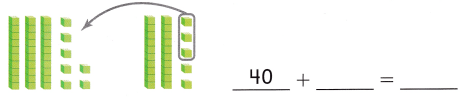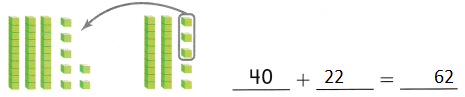Explanation:
37 + 25 = 62
40 + 22 = 62
25 is written as 22 + 3

Question 2.
27 + 46 = ?_____________ + __________ = ___________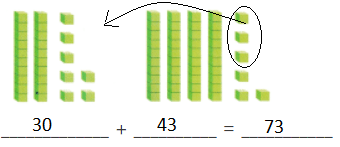Explanation:
46 is written as 43 + 3
27 + 3 = 30
30 + 43 = 73

Question 3.
14 + 29 = ?_____________ + __________ = ___________